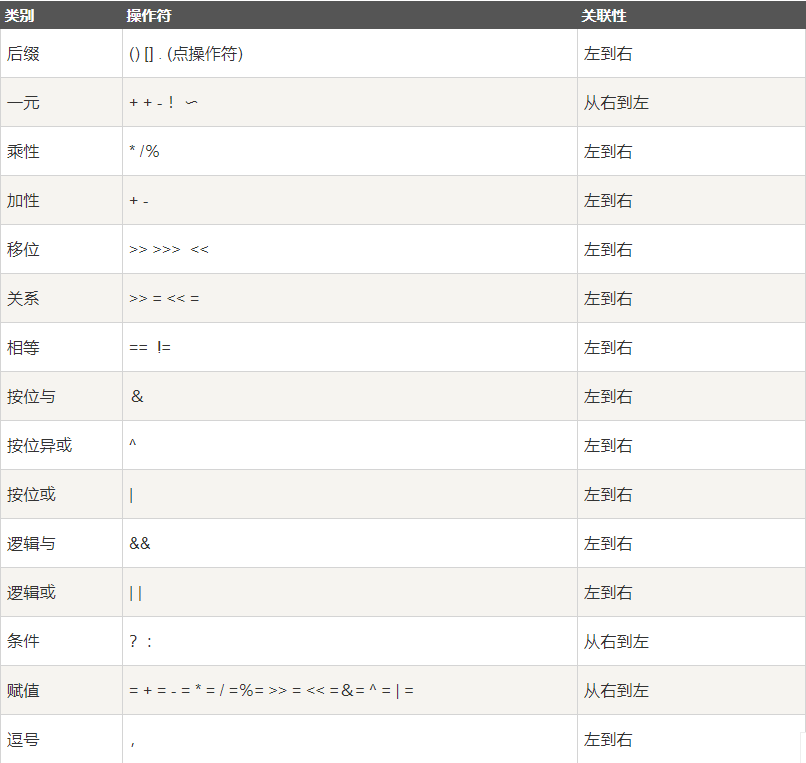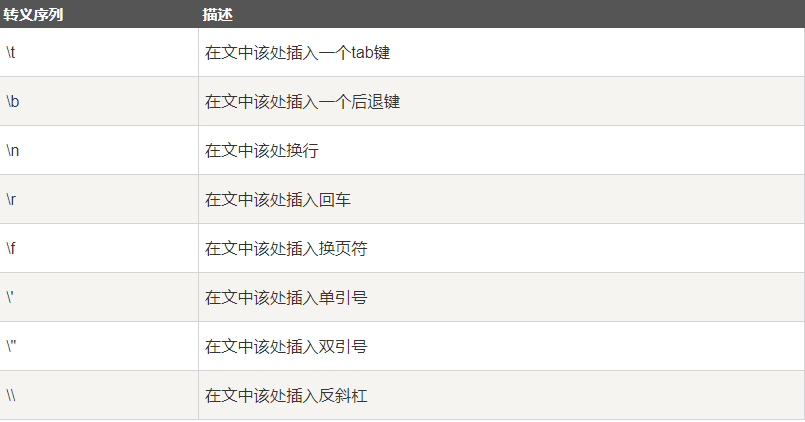# Java基础知识点总结（二）

## 1.Java运算符优先级## 2.内置数据类型的包装类

• 抽象类Number是所有包装类（Integer、Long、Byte、Double、Float、Short）的父类。

• 当数据类型要作为对象使用时，编译器会把内置类型装箱为包装类。

• 当对象要作为数据类型使用时，编译器会把包装类拆箱为内置类型。

public class Test{
public static void main(String args[]){
Integer x = 5; x = x + 10;
System.out.println(x);
}
}

## 3.Math类

• Math的方法都被定义为static形式，所以主函数可以直接调用Math类。

## 4.非访问修饰符

• static：用来修饰类方法和类变量。

• final：用来修饰类、方法和变量，final 修饰的类不能够被继承，修饰的方法不能被继承类重新定义，修饰的变量为常量，是不可修改的。

• abstract：用来创建抽象类和抽象方法。

• synchronized、volatile：主要用于线程的编程。

## 5.Number & Math 类常用方法

• ##### xxxValue()：用于将 Number 对象转换为 xxx 数据类型的值并返回。
public class Test{
public static void main(String args[]){
Integer x = 5; // 返回 byte 原生数据类型
System.out.println( x.byteValue() ); // 返回 double 原生数据类型
System.out.println(x.doubleValue()); // 返回 long 原生数据类型
System.out.println( x.longValue() );
}
}
• ##### compareTo()：用于将 Number 对象与方法的参数进行比较（两个不同类型的数据不能用此方法来比较）。
1. 返回值：

• 如果指定的数与参数相等返回0。

• 如果指定的数小于参数返回 -1。

• 如果指定的数大于参数返回 1。

2. 实例：

public class Test{ public static void main(String args[]){ Integer x = 5; System.out.println(x.compareTo(3));//运行结果为1 System.out.println(x.compareTo(5));//运行结果为0 System.out.println(x.compareTo(8));//运行结果为-1 } }
• ##### equals()：用于判断 Number 对象与方法的参数是否相等。
Integer x = 5; Integer y = 10;

System.out.println(x.equals(y));//返回false
• ##### valueOf()：用于返回给定参数的原生 Number 对象值，参数可以是原生数据类型, String等。
public class Test{ public static void main(String args[]){ Integer x =Integer.valueOf(9); Double c = Double.valueOf(5); Float a = Float.valueOf("80");

Integer b = Integer.valueOf("444",16);   // 使用 16 进制
​
System.out.println(x);
System.out.println(c);
System.out.println(a);
System.out.println(b);
}
}
• ##### toString()：用于将 Number 对象值以一个字符串返回。
public class Test{ public static void main(String args[]){ Integer x = 5;

System.out.println(x.toString());
System.out.println(Integer.toString(12));
}
}

## 6.转义序列posted @ 2019-09-05 01:42  落成  阅读(190)  评论(0编辑  收藏